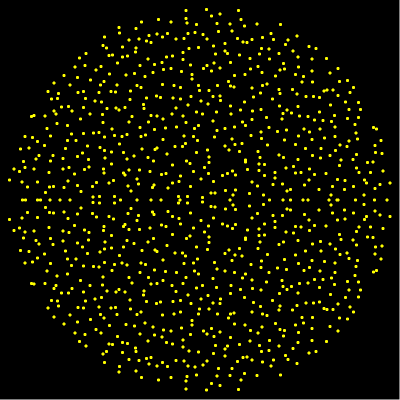M A T H 2 1 B
Mathematics Math21b Spring 2007
Linear Algebra and Differential Equations
Exhibit:In your Mathematica lab, you have explored the statistics of the eigenvalues of a large nxn random matrix. ```n=1000; A = Table[Random[]-1/2,{n},{n}]; vec[z_]:={Re[z],Im[z]}; EV=Eigenvalues[A]; Show[Graphics[Table[Point[vec[EV[[i]]]],{i,Length[EV]}]], AspectRatio->1] ``` You saw that the eigevanvalues essentially become uniformly distributed on a disc for large n. This is a variant of Girko's Circular law. Also the asymptotic distribution of random symmetric matrices is knonw. Since the eigenvalues are real, this gives a distribution on the line which is called Wigners Semicircle law.